# Accounting Ratios (Part - 5) Notes | Study TS Grewal Solutions - Class 12 Accountancy - Commerce

## Commerce: Accounting Ratios (Part - 5) Notes | Study TS Grewal Solutions - Class 12 Accountancy - Commerce

The document Accounting Ratios (Part - 5) Notes | Study TS Grewal Solutions - Class 12 Accountancy - Commerce is a part of the Commerce Course TS Grewal Solutions - Class 12 Accountancy.
All you need of Commerce at this link: Commerce

Question:101

Calculate Working Capital Turnover Ratio from the following information:

Revenue from Operations  30,00,000; Current Assets  12,50,000; Total Assets  20,00,000; Non-current Liabilities  10,00,000, Shareholders' Funds  5,00,000.
Solution: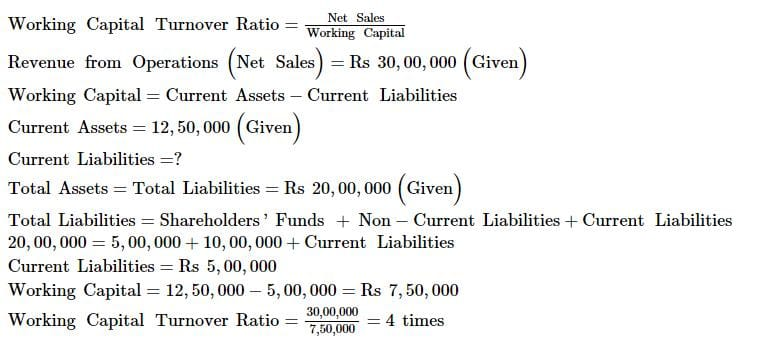Question:102

A company earns Gross Profit of 25% on cost. For the year ended 31st March, 2017 its Gross Profit was  5,00,000; Equity Share Capital of the company was  10,00,000; Reserves and Surplus  2,00,000; Long-term Loan  3,00,000 and Non-current Assets were  10,00,000.

Compute the 'Working Capital Turnover Ratio' of the company.
Working Capital Turnover Ratio= Revenue from Operation/Working Capital
Solution:

Gross Profit = 25% on Cost

Let the Cost of Goods sold be  100.

Gross Profit = 25

Revenue from Operations = 100 + 25 = 125

When Gross profit is  25, revenue from operations is = 125

And, if Gross profit is  5,00,000 then revenue from operations will be = 5,00, 000 × 125/25 =  25,00,000

Capital Employed = Shareholder’s Funds + Non-Current Liabilities

=  10,00,000 + 2,00,000 + 3,00,000

=  15,00,000

Also, Capital Employed = Non Current Assets + Working Capital

Alternatively, Working Capital = Capital Employed – Non-current Assets =  15,00,000– 10,00,000

=  5,00,000

Hence, Working Capital Turnover Ratio= 25,00,000/5,00,000= 5 times

Question:103

Compute Gross Profit Ratio from the following information:

Cost of Revenue from Operations Cost of Goods Sold

5,40,000; Revenue from Operations Net Sales

6,00,000.

Solution:

Gross Profit = Revenue from Operations – Cost of Revenue from Operations

= 6,00,000 – 5,40,000

= Rs 60,000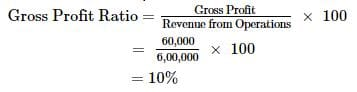Question:104

From the following, calculate Gross Profit Ratio:

Gross Profit:50,000; Revenue from Operations 5,00,000; Sales Return: 50,000.

Solution:

Net Sales = Rs 5, 00, 000Gross Profit = Rs 50, 000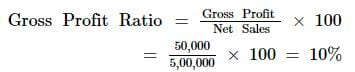Note: Here we will not deduct the amount of sales return because the amount of net sales has already been provided in the question.

Question:105

Compute Gross Profit Ratio from the following information:

Revenue from Operations, i.e., Net Sales = 4,00,000; Gross Profit 25% on Cost.

Solution: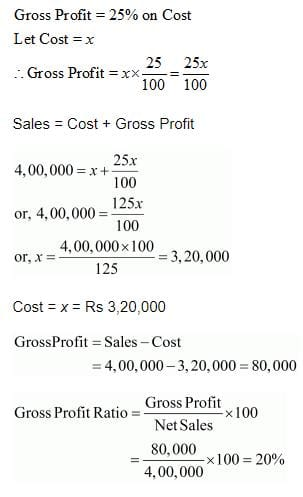Question:106

Calculate Gross Profit Ratio from the following data:

Cash Sales are 20% of Total Sales; Credit Sales are 5,00,000; Purchases are 4,00,000; Excess of Closing Inventory over Opening Inventory 25,000.
Solution:
Credit Sales = 5,00,000

Cash sales = 20% of Total Sales

Let Total Sales be ‘x’

Therefore, Cash Sales = 20% of x
Total Sales = Cash Sales + Credit Sales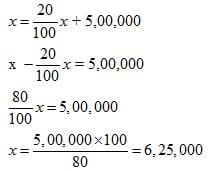Cost of Goods Sold = Purchases – Excess of Closing Stock over Opening Stock

= Rs 4,00,000 – Rs 25,000 = Rs 3,75,000

Gross Profit = Total Sales – Cost of Goods Sold

= Rs 6,25,000 – 3,75,000 = Rs 2,50,000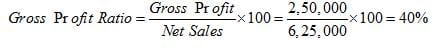Question:107

From the following information, calculate Gross Profit Ratio:

Credit Sales  5,00,000
Decrease in Inventory 10,000
Purchases 3,00,000
Returns Outward 10,000
Carriage Inwards 10,000
Wages 50,000
Rate of Credit Sale to Cash Sale 4:1

Solution:

Credit Sale = Rs 5,00,000

Rate of Credit Sale to Cash Sale = 4:1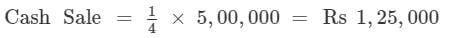Total Sales = Cash Sales + Credit Sales = Rs 1,25,000 + Rs 5,00,000 = Rs 6,25,000

Cost of Goods Sold = Purchases – Return Outward + Carriage Inwards + Wages + Decrease in Inventory

= Rs 3,00,000 – Rs 10,000 + Rs 10,000 + Rs 50,000 + Rs 10,000

= Rs 3,60,000

Gross Profit = Total Sales – Cost of Goods Sold

= Rs 6,25,000 – Rs 3,60,000 = Rs 2,65,000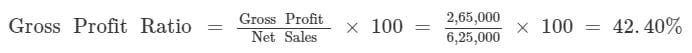Question: 108
Calculate Gross Profit Ratio from the following data:

Average Inventory 3,20,000; Inventory Turnover Ratio 8 Times; Average Trade Receivables 4,00,000; Trade Receivables Turnover Ratio 6 Times; Cash Sales 25% of Net Sales.
Solution:

Inventory Turnover Ratio = 8 times

Average Inventory = Rs 3,20,000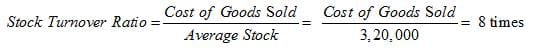Cost of Goods sold = 25,60,000

Trade Receivables Turnover Ratio = 6 times

Average Trade Receivables = Rs 4,00,000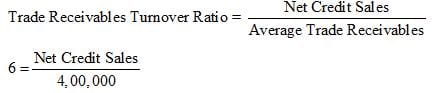Net Credit Sales = 24,00,000

Total Sales = Cash Sales + Credit Sales

Total Sales = 25% of Total Sales + Credit Sales

75% of Total Sales = 24,00,000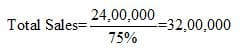Gross Profit = Total Sales – Cost of Goods Sold

= 32,00,000 – 25,60,000 = 6,40,000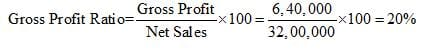Question:109

i. Revenue from Operations: Cash Sales 4,20,000; Credit Sales 6,00,000; Return 20,000. Cost of Revenue from Operations or Cost of Goods Sold 8,00,000. Calculate Gross Profit Ratio.
ii. Average Inventory 1,60,000; Inventory Turnover Ratio is 6 Times; Selling Price 25% above cost. Calculate Gross Profit Ratio.

iii. Opening Inventory 1,00,000; Closing Inventory 60,000; Inventory Turnover Ratio 8 Times; Selling Price 25% above cost. Calculate Gross Profit Ratio.
Solution: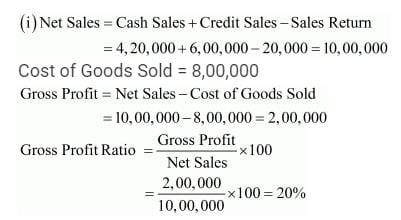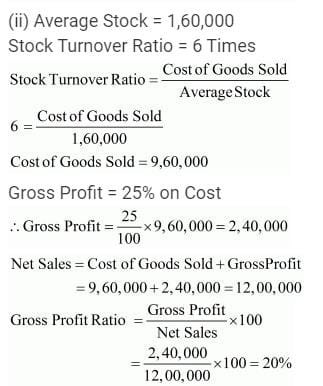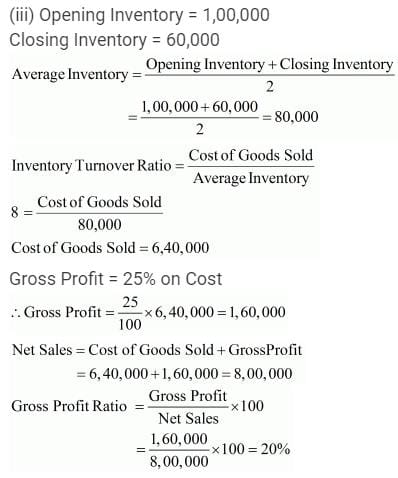Question:110

Gross Profit Ratio of a company is 25%. State giving reason, which of the following transactions will
a. increase or b. decrease or c. not alter the Gross Profit Ratio.

ii. Purchases Return 15,000.

iii. Cash Sale of Stock-in-Trade 40,000.

iv. Stock-in-Trade costing 20,000 withdrawn for personal use.

v. Stock-in-Trade costing 15,000 distributed as a free sample.

Solution: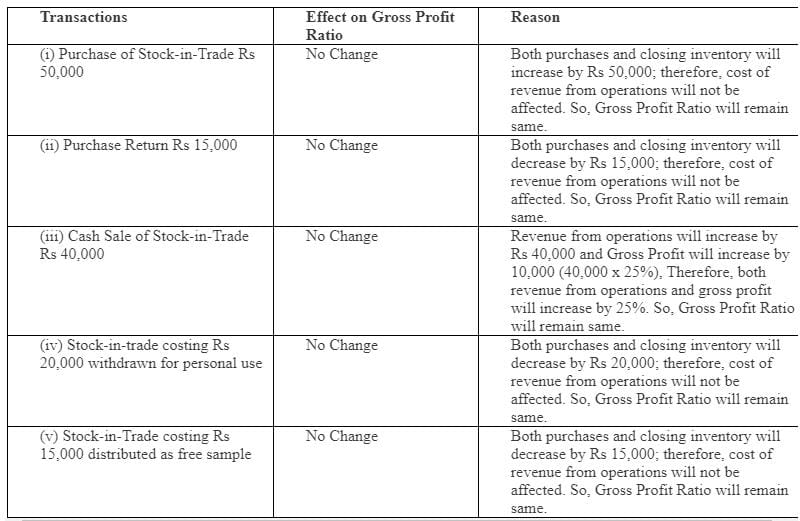Question:111

Cost of Revenue from Operations Cost of Goods Sold

3,00,000. Operating Expenses 1,20,000. Revenue from Operations: Cash Sales 5,20,000; Return 20,000. Calculate Operating Ratio.
Solution: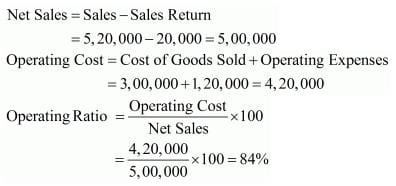Question:112

Operating Ratio 92%; Operating Expenses 94,000; Revenue from Operations 6,00,000; Sales Return 40,000. Calculate Cost of Revenue from Operations Cost of Goods Sold.
Solution: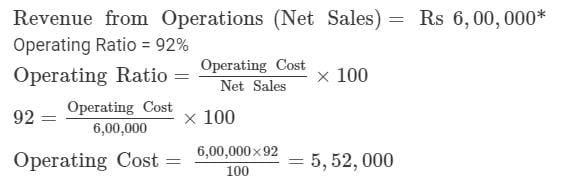Operating Cost = Cost of Goods Sold + Operating Expenses

5,52,000 = Cost of Goods Sold + 94,000

Cost of Goods Sold = Rs 4,58,000

*Note: Sales Return will not be considered since net sales are given which means sales return have already been adjusted in the sales figure.

Question:113

i. Cost of Revenue from Operations Cost of Goods Sold Rs 2,20,000; Revenue from Operations Net Sales Rs 3,20,000; Selling Expenses 12,000; Office Expenses 8,000; Depreciation 6,000. Calculate Operating Ratio.

ii. Revenue from Operations, Cash Sales 4,00,000; Credit Sales 1,00,000; Gross Profit 1,00,000; Office and Selling Expenses 50,000. Calculate Operating Ratio.
Solution: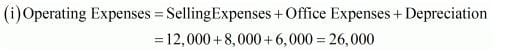Cost of Goods Sold = 2,20,000

Operating Cost = Cost of Goods Sold + Operating Expenses

Operating Cost = 2,20,000 + 26,000 = 2,46,000

Sales = 3,20,000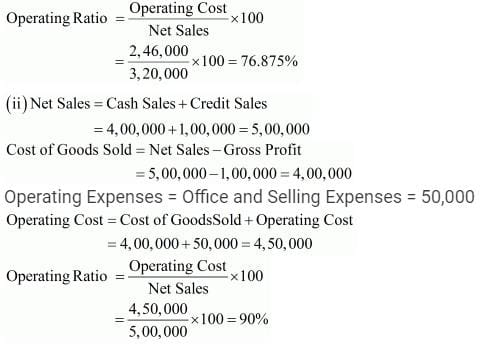Question:114

From the following information, calculate Operating Ratio:

Cost of Revenue from Operations Cost of Goods Sold 52,000
Revenue from Operation Gross Sales  88,000

Operating Expenses 18,000
Sales Return  8,000

Solution:

Net Sales = Gross Sales - Sales Return = 88, 000 - 8, 000 = Rs 80, 000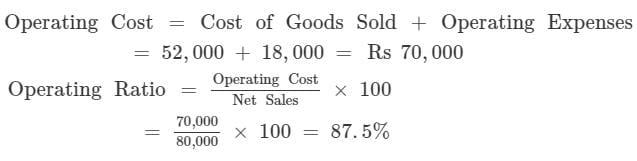Question:115

Calculate Cost of Revenue from Operations from the following information:

Revenue from Operations  12,00,000; Operating Ratio 75%; Operating Expenses  1,00,000.
Solution:
Revenue from Operations Net Sales = Rs 12, 00, 000
Operating Ratio = 75%
Operating Expenses = Rs 1, 00, 000
Find out: Cost of Revenue from Operations
Operating Ratio =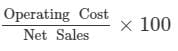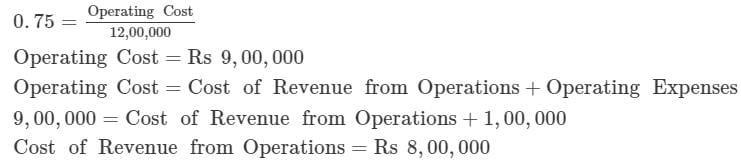Question:116

Calculate Operating Ratio from the following information:

Operating Cost  6,80,000; Gross Profit 25%; Operating Expenses  80,000.

Solution:

Given: Operating Cost = Rs 6, 80, 000
Operating Expenses = Rs 80, 000
Gross Profit Ratio = 25 %
Find out: Operating Ratio
Operating Cost = Cost of Revenue from Operations + Operating Expenses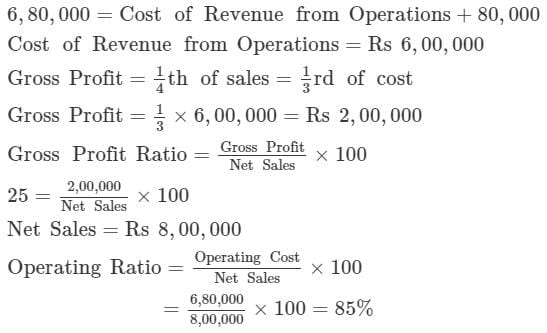Question:117

Calculate Operating Profit Ratio from the following information:

Opening Inventory 1,00,000
Closing Inventory 1,50,000

Purchases  10,00,000
Loss by fire  20,000

Revenue from Operations, i.e., Net Sales  14,70,000

Solution:

Cost of Goods Sold = Opening Inventory + Purchases – Closing Inventory

= 1,00,000 + 10,00,000 – 1,50,000 = 9,50,000

Operating Expenses = Administrative and Selling Expenses = 1,70,000

Operating Cost = Cost of Goods Sold + Operating Expenses

= 9,50,000 + 1,70,000 = 11,20,000

Net Sales = 14,70,000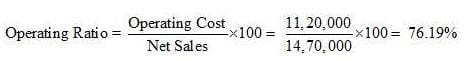Operating Profit Ratio = 100 – Operating Ratio = 100 – 76.19 = 23.81%

Question:118

Calculate Operating Profit Ratio from the Following:

Revenue from Operations Net Sales 5,00,000

Cost of Revenue from Operations Cost of Goods Sold 2,00,000

Wages 1,00,000

Interest on Borrowings 5,000

Solution:

Cost of Goods Sold = 2,00,000

Operating Expenses = Office and Administrative Expenses = 50,000

Operating Cost = Cost of Goods Sold + Operating Expenses

= 2,00,000 + 50,000 = 2,50,000

Net Sales = 5,00,000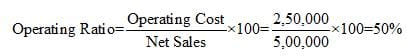Operating Profit Ratio = 100 – Operating Ratio = 100 – 50 = 50%

Question:119

What will be the Operating Profit Ratio, if the Operating Ratio is 82.59%?

Solution:

Operating Ratio = 82.59%

Operating Ratio + Operating Profit Ratio = 100%

Operating Profit Ratio = 100% − 82.59% = 17.41%

Question:120

Calculate Operating Profit Ratio,in each of the following alternative cases:

Case 1: Revenue from Operations Net Sales 10,00,000; Operating Profit  1,50,000.

Case 2: Revenue from Operations Net Sales 6,00,000; Operating Cost  5,10,000.

Case 3: Revenue from Operations Net Sales 3,60,000; Gross Profit 20% on Sales; Operating Expenses  18,000

Case 4: Revenue from Operations Net Sales  4,50,000; Cost of Revenue from Operations  3,60,000; Operating Expenses  22,500.

Case 5: Cost of Goods Sold, i.e., Cost of Revenue from Operations  8,00,000; Gross Profit 20% on Sales; Operating Expenses  50,000.
Solution: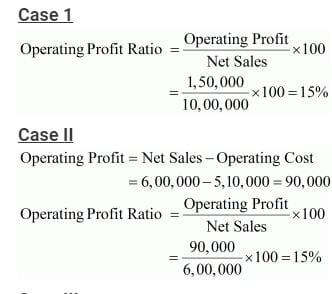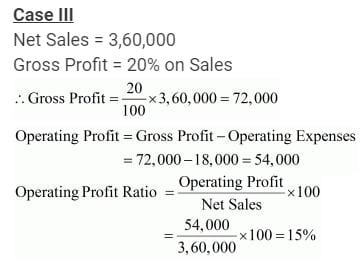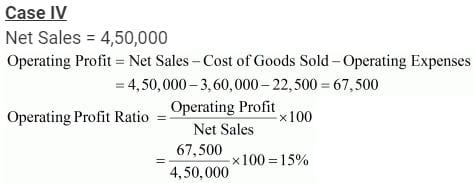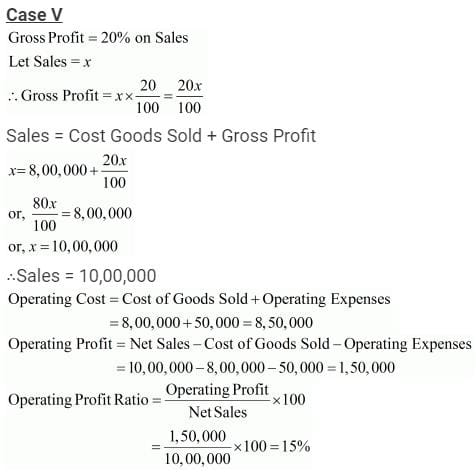Question:121

Revenue from Operations  9,00,000; Gross Profit 25% on Cost; Operating Expenses  45,000. Calculate Operating Profit Ratio.

Solution: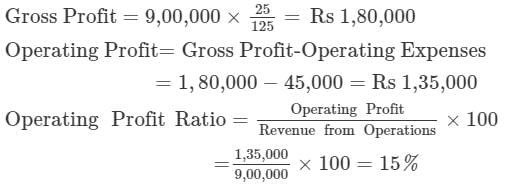Question:122

Operating Cost  3,40,000; Gross Profit Ratio 20%; Operating Expenses  20,000. Calculate Operating Profit Ratio.

Solution:

Cost of Revenue from Operations = Operating Cost - Operating Expenses  = 3,40,000 - 20,000 = Rs 3,20,000 3,20,000 × 20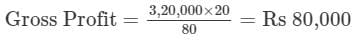Revenue from Operations = Cost of Revenue from Operations + Gross Profit

=3,20,000+80,000 = Rs 4,00,000

Operating Profit = Revenue from Operations - Operating Cost

= 4, 00, 000 − 3, 40, 000 = Rs 60,000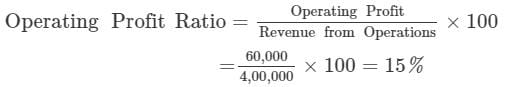Question:123

Cash Sales  2,20,000; Credit Sales  3,00,000; Sales Return  20,000; Gross Profit  1,00,000; Operating Expenses  25,000; Non-operating incomes  30,000; Non-operating Expenses  5,000. Calculate Net Profit Ratio.

Solution: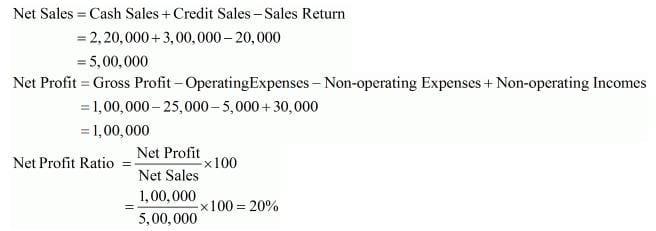Question:124

Revenue from Operations, i.e., Net Sales  6,00,000. Calculate Net Profit Ratio.

Solution:
Net Sales = 6,00,000

Net profit = 60,000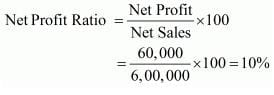Question:125

Revenue from Operations, i.e., Net Sales  8,20,000; Return  10,000; Cost of Revenue from Operations Cost of Goods Sold  5,20,000; Operating Expenses  2,09,000; Interest on Debentures  40,500; Gain Profit on Sale of a Fixed Asset  81,000. Calculate Net Profit Ratio.

Solution:

Net Sales = Rs 8, 20, 000

Gross Profit = Net Sales - Cost of Goods Sold
= 8, 20, 000 - 5, 20, 000
= Rs 3, 00, 000

Net Profit = Gross Profit - Operating Expenses - Interest on Debentures + Profit on Sale of Fixed Asset
= 3, 00, 000 - 2, 09, 000 - 40, 500 + 81, 000
= Rs 1, 31, 500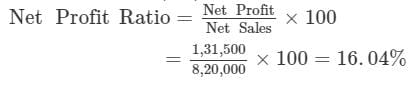Question:126
Revenue from Operations 4,00,000; Gross Profit Ratio 25%; Operating Ratio 90%. Non-operating Expenses 2,000; Non-operating Income 22,000. Calculate Net Profit Ratio.
Solution:
Net Profit = Operating Profit + Non Operating Incomes - Non Operating Expenses

= 40,000+22,000- 2,000 = Rs 60,000

Operating Profit Ratio = 100 − Operating Ratio =100-90 =10%

Operating Profit = 4, 00, 000 × 10% = Rs 40,000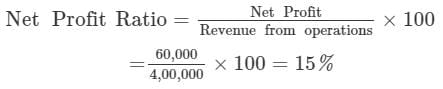Question:127

Calculate Return on Investment ROI

from the following details: Net Profit after Tax  6,50,000; Rate of Income Tax 50%; 10% Debentures of  100 each  10,00,000; Fixed Assets at cost  22,50,000; Accumulated Depreciation on Fixed Assets up to date  2,50,000; Current Assets  12,00,000; Current Liabilities  4,00,000.

Solution:

Net Fixed Assets = Fixed Assets at cost

− Accumulated Depreciation

= 22,50,000 − 2,50,000 = 20,00,000

Capital Employed = Net Fixed Assets + Current Assets − Current Liabilities

= 20,00,000 + 12,00,000 − 4,00,000

= 28,00,000

Interest on 10% Debentures = 10% of 10,00,000 = 1,00,000

Let Profit before Tax be = x

Profit after Tax = Profit Before Tax − Tax

Tax Rate = 50%

∴ Tax = 0.5 x

x − 0.5 x = 6,50,000

x = 13,00,000

Net Profit before Tax = x = 13,00,000

Profit before Interest and Tax = Profit before Tax + Interest on Long-term Debt

= 13,00,000 + 1,00,000

= 14,00,000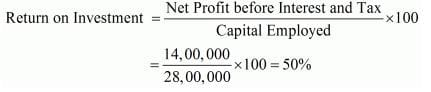Question:128

Net Profit before Interest and Tax 2,50,000; Capital Employed 10,00,000. Calculate Return on Investment.
Solution:

Net Profit before Interest and Tax = 2,50,000

Capital Employed = 10,00,000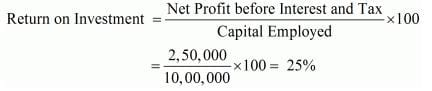Question:129

Net Profit before Interest and Tax 6,00,000; Net Fixed Assets 20,00,000; Net Working Capital 10,00,000; Current Assets 11,00,000. Calculate Return on Investment.
Solution:

Net Profit before Interest and Tax = 6,00,000

Capital Employed = Net Fixed Assets + Net Working Capital

= 20,00,000 + 10,00,000 = 30,00,000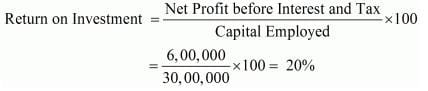Question:130

Net Profit before Interest and Tax 4,00,000; 15% Long-term Debt 8,00,000; Shareholders' Funds 4,00,000. Calculate Return on Investment.

Solution:

Net Profit before Interest and Tax = 4,00,000

Capital Employed = 15% long-term Debt + Shareholders’ Funds

= 8,00,000 + 4,00,000 = 12,00,000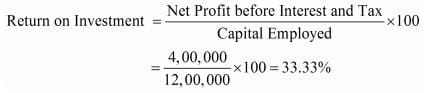Question:131

y Ltd.'s profit after interest and tax was  1,00,000. Its Current Assets were  4,00,000; Current Liabilities  2,00,000; Fixed Assets  6,00,000 and 10% Long-term Debt  4,00,000. The rate of tax was 20%. Calculate 'Return on Investment' of Y Ltd.

Solution:

Return on Investment = Net Profit before Interest, Tax and Dividend/Capital Employed × 100

Let Profit before tax be Rs 100

Tax = Rs 20

Profit after tax = 100 – 20 = 80

If Profit after tax is Rs 80 then profit before tax is = Rs 100

If Profit after tax is Rs 1,00,000 then profit before tax is = Rs 1, 00, 000 × 100/80 = 1,25,000

Interest on long-term borrowings = Rs 4, 00, 000 × 10/100 = Rs 40,000

Profit after interest and Tax = Rs 1,25,000 + 40,000 = Rs 1,65,000

Capital Employed = Fixed Assets + Current Assets – Current Liabilities

=  6,00,000 + 4,00,000 – 2,00,000

= 8,00,000

Return on Investment = 1,65,000/8,00,000 × 100 = 20.625% or 20.63%

approx.

Question:132

From the following Balance Sheet of Global Ltd., you are required to calculate Return on Investment for the year 2018-19: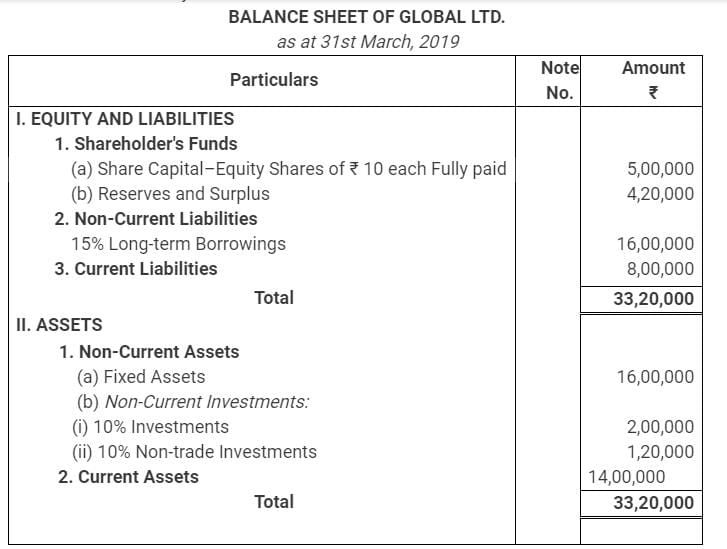Solution:
Return on Investment = (Net Profit before Interest, Tax and Dividend/ Capital Employed × 100)

Interest on borrowings = ₹ (16,00,000 × 15/100)= ₹ 2,40,000

Net Profit before Tax = ₹ 9,72,000

Net Profit before Interest and Tax = ₹ (9,72,000 + 2,40,000) = ₹ 12,12,000

Net Profit before Interest and Tax (excluding interest on Non-trade investments) = ₹ (12,12,000 – 12,000) = ₹ 12,00,000

Capital Employed = Shareholder’s Funds + Non-Current Liabilities – Non-Trade Investment

= ₹ (5,00,000 + 4,20,000 + 16,00,000 – 1,20,000) = ₹ 24,00,000

Return on Investment = (12,00,000/24,00,000 × 100) = 50%

Question:133

Following is the Balance Sheet of the Bharati Ltd. as at 31st March, 2019: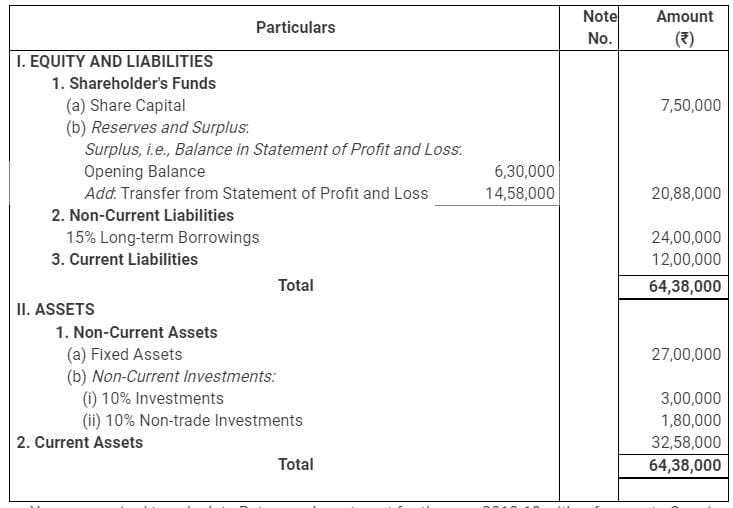You are required to calculate Return on Investment for the year 2018-19 with reference to Opening Capital Employed.

Solution:
Return on Investment = (Net Profit before Interest, Tax and Dividend/ Capital Employed × 100)

Interest on borrowings = ₹ (24,00,000 × 15/100) = ₹3,60,000

Net Profit before Interest and Tax = Net Profit after tax + Interest on borrowings – Interest received on Non-trade Investments

= ₹ (14,58,000 + 3,60,000 – 18,000) = ₹ 18,00,000

Opening Capital Employed = Shareholder’s Funds (Opening) + Non-Current Liabilities (Opening) – Non-Trade Investment

= ₹(7,50,000 + 6,30,000 + 24,00,000 – 1,80,000) = ₹36,00,000

Return on Investment = (18,00,000/36,00,000 × 100) = 50%

Question:134
State with reason whether the following transactions will increase, decrease or not change the 'Return on Investment' Ratio:

(i) Purchase of machinery worth ₹10,00,000 by issue of equity shares.

(ii) Charging depreciation of ₹25,000 on machinery.

(iii) Redemption of debentures by cheque ₹2,00,000.

(iv) Conversion of 9% Debentures of ₹1,00,000 into equity shares.
Solution: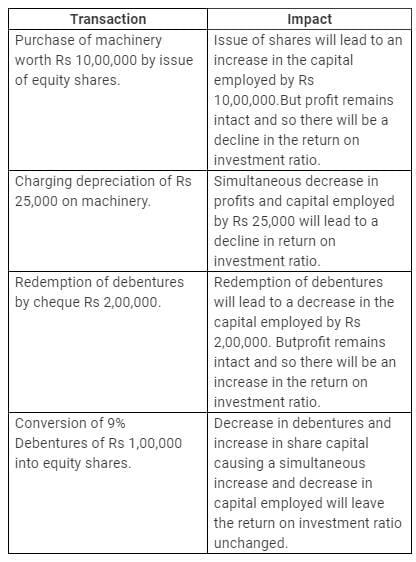Question:135
Opening Inventory ₹80,000; Purchases ₹4,30,900; Direct Expenses ₹4,000; Closing Inventory ₹1,60,000; Administrative Expenses ₹21,100; Selling and Distribution Expenses ₹40,000; Revenue from Operations, i.e., Net Sales ₹10,00,000. Calculate Inventory Turnover Ratio; Gross Profit Ratio; and Opening Ratio.
Solution:
(i) Opening Inventory = 80,000

Closing Inventory = 1,60,000

Cost of Goods Sold = Opening Inventory + Purchases + Direct Expenses − Closing Inventory

= 80,000 + 4,30,900 + 4,000 − 1,60,000

= 3,54,900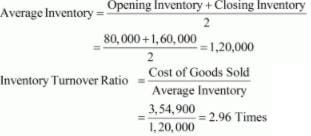(ii) Sales = 10,00,000

Gross Profit = Net Sales − Cost of Goods Sold

= 10,00,000 − 3,54,900 = 6,45,100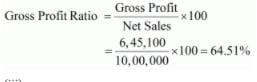(iii) Operating Expenses = Administration Expenses + Selling and Distribution Expenses

= 21,100 + 40,000 = 61,100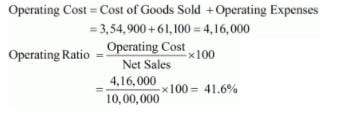Question:136

Following information is given about a company: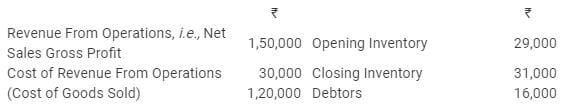From the above information, calculate following ratios:

(i) Gross Profit Ratio,

(ii) Inventory Turnover Ratio, and

Solution:
(i) Sales = 1,50,000

Gross Profit = 30,000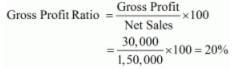(ii) Opening Inventory = 29,000

Closing Inventory = 31,000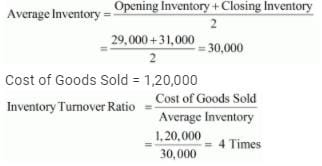(iii)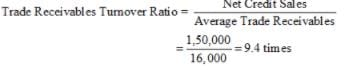Question:137
From the following information, calculate any two of the following ratios:

(i) Current Ratio;

(ii) Debt to Equity Ratio; and

(iii) Operating Ratio.

Revenue from Operations (Net Sales) ₹ 1,00,000; cost of Revenue from Operations (Cost of Goods Sold) was 80% of sales; Equity Share Capital ₹ 7,00,000; General Reserve ₹ 3,00,000; Operating Expenses ₹ 10,000; Quick Assets ₹ 6,00,000; 9% Debentures ₹ 5,00,000; Closing Inventory ₹ 50,000; Prepaid Expenses ₹ 10,000 and Current Liabilities ₹ 4,00,000.
Solution:
(i) Current Assets = Quick Assets + Closing Stock + Prepaid Expenses

= 6,00,000 + 50,000 + 10,000 = 6,60,000

Current Liabilities = 4,00,000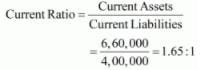(ii) Long-term Debts = 9% Debentures = 5,00,000

Shareholder’s Funds = Equity Share Capital + General Reserve

= 7,00,000 + 3,00,000 = 10,00,000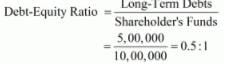(iii) Sales = 1,00,000

Cost of Goods Sold = 80% of Sales = 80,000

Operating Expenses = 10,000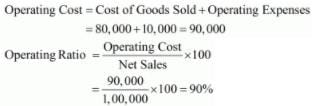Question:138
From the following information, calculate Inventory Turnover Ratio; Operating Ratio and Working Capital Turnover Ratio:

Opening Inventory ₹ 28,000; Closing Inventory ₹ 22,000; Purchases ₹ 46,000; Revenue from Operations,  i.e., Net Sales ₹ 80,000; Return ₹10,000; Carriage Inwards ₹ 4,000; Office Expenses ₹ 4,000; Selling and Distribution Expenses ₹ 2,000; Working Capital ₹ 40,000.
Solution:
(i) Opening Inventory = 28,000

Closing Inventory = 22,000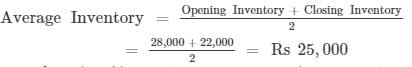Cost of Goods Sold = Opening Inventory + Purchases + Carriage Inwards − Closing Inventory

= 28,000 + 46,000 + 4,000 − 22,000 = 56,000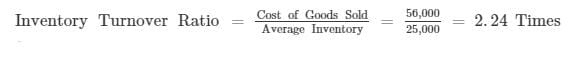(ii) Operating Expenses = Office Expenses + Selling and Distribution Expenses

= 4,000 + 2,000 = 6,000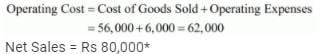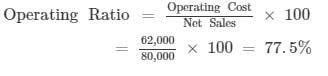(iii) Working Capital = 40,000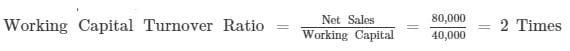*Note: Sales return will not be considered as the amount of net sales is provided in the question.

Question:139
From the following calculate:

(a) Current Ratio; and
(b) Working Capital Turnover Ratio.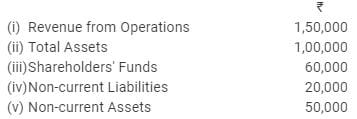Solution: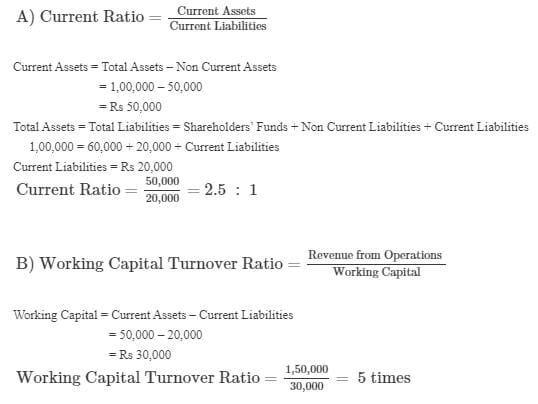Question:140
Calculate following ratios on the basis of the following information:

(i) Gross Profit Ratio;

(ii) Current Ratio;

(iii) Acid Test Ratio; and

(iv) Inventory Turnover Ratio.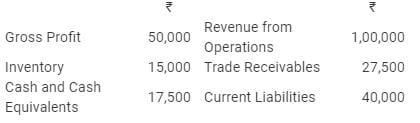Solution: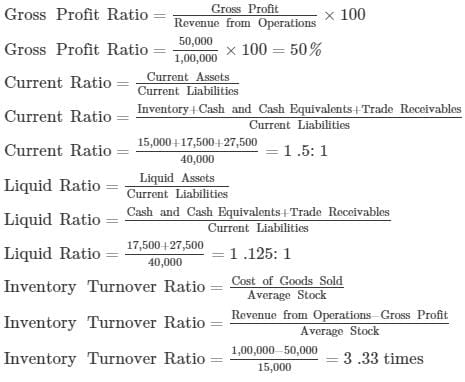Question:141
Calculate following ratios on the basis of the given information:

(i) Current Ratio;

(ii) Acid Test Ratio;

(iii) Operating Ratio; and

(iv) Gross Profit Ratio.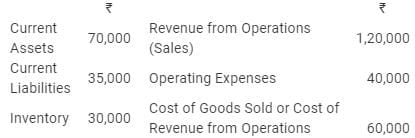Solution:
(i) Current Assets = 70,000

Current Liabilities = 35,000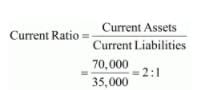(ii) Liquid Assets = Current Assets − Inventory

= 70,000 − 30,000 = 40,000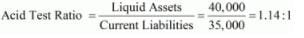(iii) Net Sales = 1,20,000

Operating Cost = Cost of Goods Sold + Operating Expenses

= 60,000 + 40,000 = 1,00,000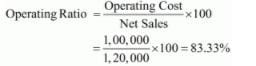(iv) Gross Profit = Net Sales − Cost of Goods Sold

= 1,20,000 − 60,000 = 60,000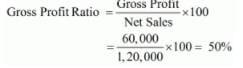Question:142
From the information given below, calculate any three of the following ratio:

(i) Gross Profit Ratio;

(ii) Working Capital Turnover Ratio:

(iii) Debt to Equity Ratio; and

(iv) Proprietary Ratio.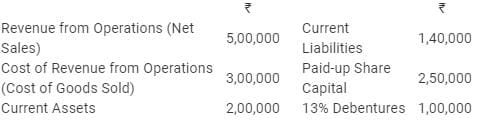Solution:
(i) Net Sales = 5,00,000

Cost of Goods Sold = 3,00,000

Gross Profit = Net Sales − Cost of Goods Sold

= 5,00,000 − 3,00,000 = 2,00,000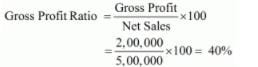(ii) Current Assets = 2,00,000

Current Liabilities = 1,40,000

Working Capital = Current Assets − Current Liabilities

= 2,00,000 − 1,40,000 = 60,000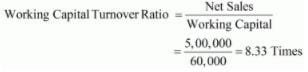(iii) Long-term Debts = 13% Debentures = 1,00,000

Equity = Paid-up Share Capital = 2,50,000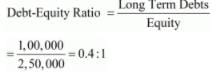(iv) Total Assets = Total Liabilities

= Current Liabilities + Paid-up Share Capital + 13% Debentures

= 1,40,000 + 2,50,000 + 1,00,000

= 4,90,000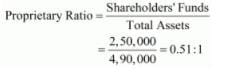Question:143
On the basis of the following information calculate:

(i) Debt to Equity Ratio; and

(ii) Working Capital Turnover Ratio.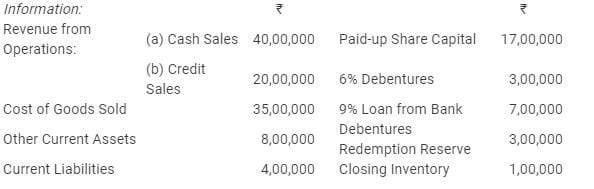Solution:
(i) Long-term Debts = 6% Debentures + 9% Loan from Bank

= 3,00,000 + 7,00,000 = 10,00,000

Equity = Paid-up Share Capital + Debenture Redemption Reserve

= 17,00,000 + 3,00,000 = 20,00,000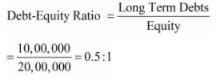(ii) Current Assets = Other Current Assets + Inventory

= 8,00,000 + 1,00,000

= 9,00,000

Working Capital = Current Assets − Current Liabilities

= 9,00,000 − 4,00,000

= 5,00,000

Net Sales = Cash Sales + Credit sales

= 40,00,000 + 20,00,000

= 60,00,000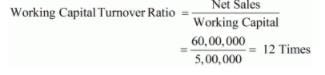Question:144
From the following, calculate (a) Debt to Equity Ratio; (b) Total Assets to Debt Ratio; and (c) Proprietary Ratio: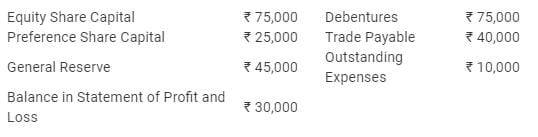Solution: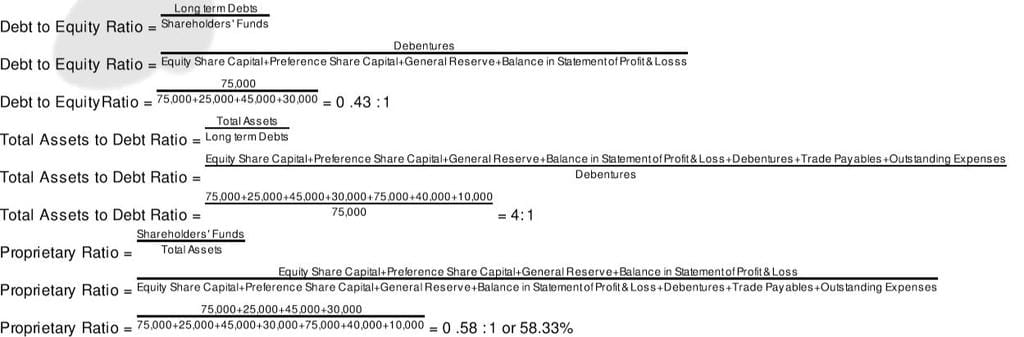Question:145

From the following information related to Naveen Ltd., calculate (a) Return on Investment and (b) Total Assets to Debt Ratio:

Information: Fixed Assets ₹ 75,00,000; Current Assets ₹ 40,00,000; Current Liabilities ₹ 27,00,000; 12% Debentures ₹ 80,00,000 and Net Profit before Interest, Tax and Dividend ₹ 14,50,000.
Solution: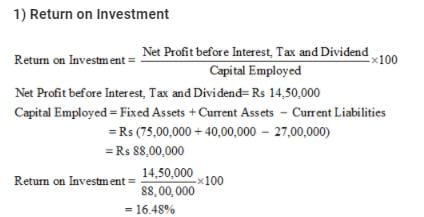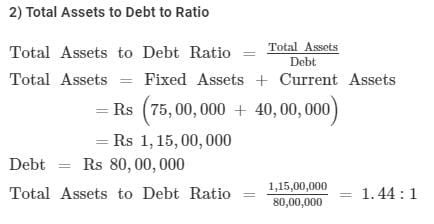Question:146
Calculate Current Ratio, Quick Ratio and Debt to Equity Ratio from the figures given below: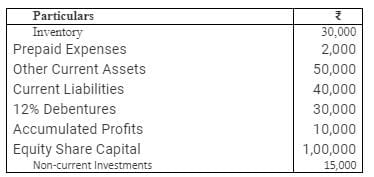Solution:
(i) Current Assets = Inventory + Prepaid Expenses + Other Current Assets

= 30,000 + 2,000 + 50,000 = 82,000

Current Liabilities = 40,000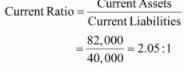(ii) Liquid Assets = Current Assets − Inventory − Prepaid Expenses

= 82,000 − 30,000 − 2,000 = 50,000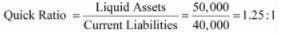(iii) Long-term Debts = 12% Debentures = 30,000

Equity = Accumulated Profits + Equity Share Capital

= 10,000 + 1,00,000 = 1,10,000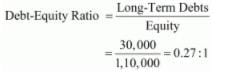Question:147
From the following informations, calculate Return on Investment (or Return on Capital Employed):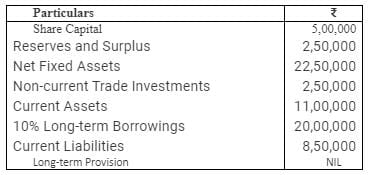Solution:
Net Profit before tax = 6,00,000

Net Profit before interest, tax and dividend = Net Profit before tax + Interest on long-term borrowings

= 6,00,000 + 10% of 20,00,000 = 6,00,000 + 2,00,000 = 8,00,000

Capital Employed = Share Capital + Reserves and Surplus + Long-term borrowings
= 5,00,000 + 2,50,000 + 20,00,000 = 27,50,000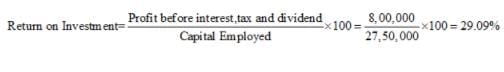The document Accounting Ratios (Part - 5) Notes | Study TS Grewal Solutions - Class 12 Accountancy - Commerce is a part of the Commerce Course TS Grewal Solutions - Class 12 Accountancy.
All you need of Commerce at this link: CommerceUse Code STAYHOME200 and get INR 200 additional OFF

## TS Grewal Solutions - Class 12 Accountancy

52 docs

Track your progress, build streaks, highlight & save important lessons and more!

,

,

,

,

,

,

,

,

,

,

,

,

,

,

,

,

,

,

,

,

,

;